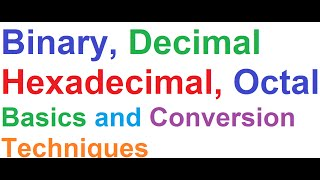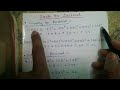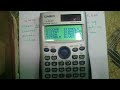# Computer Number Systems(Binary,Octal,Hexa,Decimal) Basics & Conversion Techniques + 7 Golden Rules

Apr 10, 2013

### Thumbs### Description

Computer Science & Engineering(CSE) Tutorials-
http://goo.gl/Z2hQDW

Today I'll tell you How To Convert from any base Number System to any other base number system without the help of calculator! Including the fraction conversion,also tell you 7 Golden Rules needed for those conversions. See the details description below.

Topics covered-
1. Basics of 4 types of number systems(Decimal,Binary,Octal,Hexadecimal)

2. Decimal to Other Number Systems Conversions
i) Decimal to Binary Conversion
ii) Shortcut (Weighted Method) conversion for Decimal to Binary
iii) Decimal to Octal Conversion
v) Golden Rule #1

3. Back To Decimal Conversion
i) Binary To Decimal Conversion
ii) Octal To Decimal Conversion
iv) Golden Rule #2

4. Converting With The Fractions
i) Decimal Fraction to Binary Fraction
ii) Decimal Fraction to Octal Fraction
iii) Golden Rule #3

5. From Other Bases Fraction to Back To Decimal
i) Octal Fraction to Decimal Fraction
ii) Binary Fraction to Decimal Fraction
iii) Golden Rule #4

6. Interconversions Between The Bases
i) Binary to Octal Direct Conversion(without going through Decimal)
ii) Binary to Hexadecimal Direct Conversion(without going through Decimal)
iii) Golden Rule #5

7. Some Logical Questions
i) Why Grouping of 3 Binary Bits for Octal Conversion?
ii) Why Grouping of 4 Binary Bits for Hexadecimal Conversion?

8. Golden Rule #6
9. Golden Rule #7

I make videos on Statistics,Numerical Methods,
Business & Financial Mathematics,Operation Research,Computer Science & Engineering(CSE),Android Application Reviews,India Travel & Tourism,Street Foods,Life Tips and many other topics.